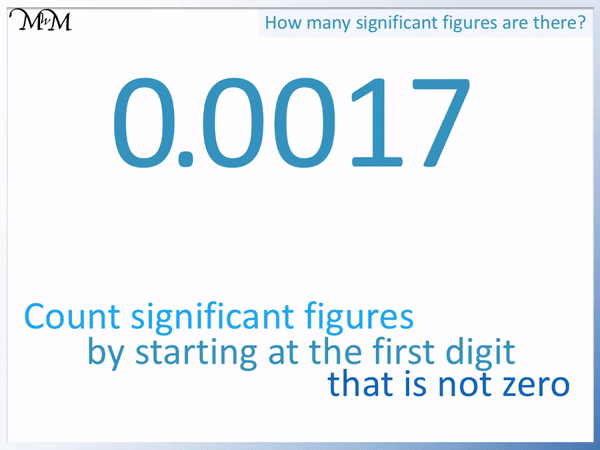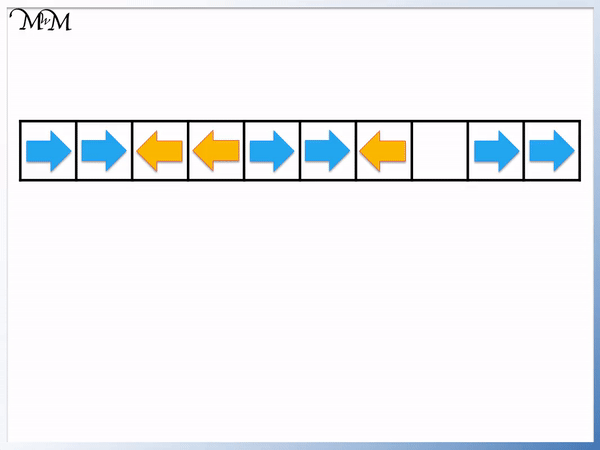# Counting Significant Figures

Counting Significant Figures• Significant figures are counted from the first digit that is not a zero when reading from left to right.
• The first digit in 0.10205 is a zero and so we do not count it.
• The next digit after the zero is a 1.
• This is not zero and so, we start counting.
• 1 is the first significant figure of 0.10205.
• Once we have started counting significant figures, we continue to count all digits including any zeros.
• The 0 is the 2nd significant figure.
• The 2 is the 3rd significant figure
• The 0 is the 4th significant figure.
• The 5 is the 5th significant figure.
• There are 5 significant figures in 0.10205 .

Count significant figures by starting at the first digit that is not zero when reading from left to right.• We count significant figures by starting at the first digit that is not zero when reading from left to right.
• The first digit is 9. It is not zero so we start counting.
• 9 is the 1st significant figure.
• 1 is the second significant figure.
• We have begun counting, so we count the zeros that come after the 9 and the 1.
• 91000 contains 5 significant figures.# How to Determine the Number of Significant Figures in a Number

## What are Significant Figures?

Significant figures are the first digit of a number that is not zero, along with all of the digits that come after it, when reading from left to right.

To determine the number of significant figures in a number, look at each digit from left to right. Start counting the digits of the number from the first digit that is not a zero. Once you have started counting, count every digit until you get to the end, even if these digits are zeros.

Here is an example.We read the digits from left to right.

The first digit before the decimal point is a zero and so we ignore it. We don’t start counting yet.

The next digit is a 1. This is not zero so we start counting. The first significant figure is 1.

Because we have now started counting, we count all of the following digits too, including any zeros.

The 2nd significant figure is 0.

The 3rd significant figure is 2.

The 4th significant figure is 0.

The 5th significant figure is 5.

It is important to understand that we don’t count any zeros at the start of a number but we do count all zeros that come after any digit that is not zero.

Significant figures are important when dealing with very large or very small numbers. Significant figures are the important digits in a number that allow us to compare two numbers.

If we know how to count significant figures we can also round numbers to a certain degree of accuracy. Rounding is useful when dealing with very large numbers or decimal numbers so we do not have to write every digit.

## Significant Figures with Decimals

In these examples we will look at counting the significant figures of decimal numbers.

Remember that we do not start counting until we get to a number that is not zero.

This means that all zeros at the start of a decimal number are insignificant and are ignored when counting significant figures.

Here is 0.00527.

We have three zeros at the start of this number. One zero before the decimal point and two after it. We ignore all three.

The digit of ‘5’ is the first digit that is not a zero and so it is the first significant figure.2 is the second significant figure and 7 is the third.

We have ignored the zeros and counted the 3 significant figures in the ‘527’ part of the number 0.00527.

0.00527 has three significant figures.

Here is another example of counting the significant figures in a decimal number. We have 0.0017.

Again this decimal number has leading zeros, which we can ignore.

We first start counting at the first digit that is not a zero: 1.The second significant figure is 7.

The number 0.0017 has two significant figures.

In this example we have a decimal number with no zeros. We will count the significant figures in the number 5.388.5 is the first digit when read from left to right and it is not a zero. This means that it is significant and we start counting immediately.

5 is the first significant figure.

3 is the second significant figure.

8 is the third significant figure.

8 is the fourth significant figure.

5.388 has 4 significant figures.

## Significant Figures with Whole Numbers

We will now count significant figures in whole numbers.

Counting significant figures in whole numbers is very easy.

The number of significant figures in a whole number is simply the number of digits shown in the number.

Our first example is 324.

Remember that we start counting from the first digit that is not a zero.3 is the first significant figure. 2 is the second significant figure and 4 is the third significant figure.

324 has three significant figures. Because 324 is a whole number we can simply count its digits to see how many significant figures it has.

Here is another example of counting significant figures with whole numbers.

We will count the number of significant figures in 91000.9 is not a zero so we start counting the digits immediately.

We also count the zeros in this number because they come after the 9 and the 1. We have start counting significant figures with the 9 and once we have started counting, we don’t stop.

91000 has 5 significant figures.

Again, because 91000 is a whole number, we just count how many digits it contains, which is 5.

## Significant Figures with Zero

It is important to bear in mind some special cases involving the digit of zero.

The number 5 has 1 significant figure. The number 5.0 has two significant figures.

Even though 5 is the same value as 5.0, the number is written differently.

5.00 would have three significant figures. If the number is written like this, we assume it is written like this because the zeros are important and so, they are significant.

Likewise, the number 100 has 3 significant figures but the number 100.000 has 6 significant figures.

We will now look at the number zero.

The number 0 has one significant figure.

Normally, we say that we only start counting significant figures from the first digit that is not a zero. However 0 is the only digit in this example.

We might say that if it wasn’t significant, we wouldn’t write it at all. Because we have written a 0, then it is significant to show the value of the number zero.

The number zero is the only number that we need to make an exception for,Now try our lesson on Repeating Shape Patterns where we learn how to find missing shapes in different patterns.